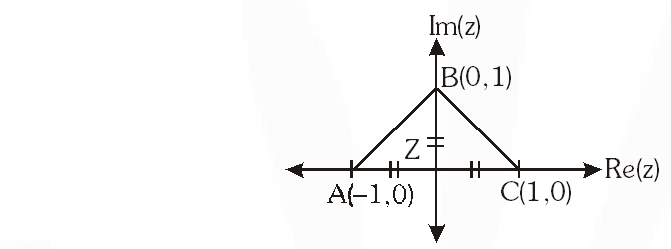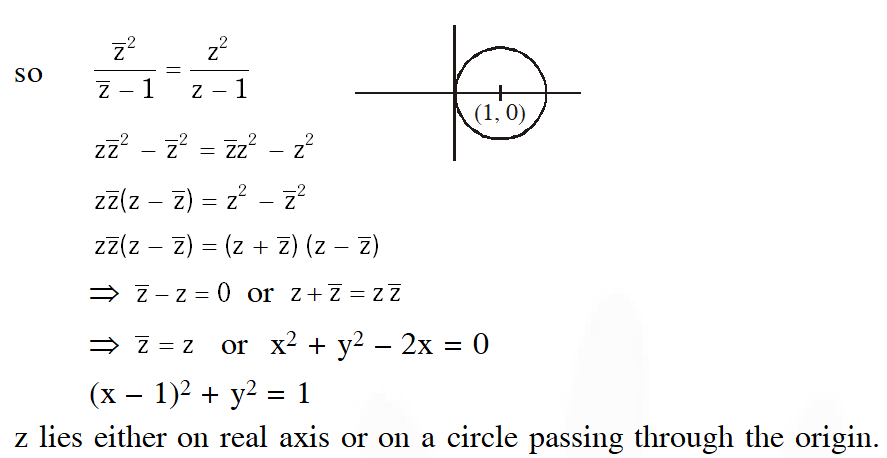Most Affordable JEE | NEET | 8,9,10 Preparation by Kota's Top IITian Doctor Faculties

# Complex Number - JEE Main Previous Year Question with Solutions`
JEE Main Previous Year Question of Math with Solutions are available at eSaral. Practicing JEE Main Previous Year Papers Questions of mathematics will help the JEE aspirants in realizing the question pattern as well as help in analyzing weak & strong areas. Get detailed Class 11th &12th Physics Notes to prepare for Boards as well as competitive exams like IIT JEE, NEET etc. eSaral helps the students in clearing and understanding each topic in a better way. eSaral is providing complete chapter-wise notes of Class 11th and 12th both for all subjects. Besides this, eSaral also offers NCERT Solutions, Previous year questions for JEE Main and Advance, Practice questions, Test Series for JEE Main, JEE Advanced and NEET, Important questions of Physics, Chemistry, Math, and Biology and many more. Download eSaral app for free study material and video tutorials.
Q. If $\left|Z-\frac{4}{Z}\right|=2,$ then the maximum value of $|Z|$ is equal to :-. (1) 2 (2) $2+\sqrt{2}$ (3) $\sqrt{3}+1$ (4) $\sqrt{5}+1$ [AIEEE -2009]
Ans. (4) $\left|z-\frac{4}{z}\right| \geq|z|-\left|\frac{4}{z}\right|$ $2 \geq|z|-\frac{4}{|z|}$ $2|z| \geq|z|^{2}-4$ $|z|^{2}-2|z|-4 \leq 0$ $|z| \leq \sqrt{5}+1$
Q. The number of complex numbers z such that $|z-1|=|z+1|=|z-i|$ equals :- (1) 0 (2)1 (3) 2 (4) $\infty$ [AIEEE -2010]
Ans. (2) z is the circumcentre (0, 0) of triangle ABC so their exist only one complex number.Q. Let $\alpha, \beta$ be real and $z$ be a complex number. If $z^{2}+\alpha z+\beta=0$ has two distinct roots on the line $\operatorname{Re} z=1,$ then it is necessary that :- (1) $|\beta|=1$ (2) $\beta \in(1, \infty)$ (3) $\beta \in(0,1)$ (4) $\beta \in(-1,0)$ [AIEEE -2011]
Ans. (2) Let $z^{2}+\alpha z+\beta=0$ has $\left(1+i y_{1}\right)$ and $\left(1+i y_{2}\right)$ so $z_{1} z_{2}=\beta$ $\left(1+i y_{1}\right)\left(1+i y_{2}\right)=\beta$ $\beta=1-\mathrm{y}_{1} \mathrm{y}_{2}+\mathrm{i}\left(\mathrm{y}_{1}+\mathrm{y}_{2}\right)(\because \beta \text { is purely real })$ here $\mathrm{y}_{1}+\mathrm{y}_{2}=0$ $\mathrm{y}_{1}=-\mathrm{y}_{2}$ $\beta=1-\mathrm{y}_{1} \mathrm{y}_{2}$ $\beta=1+\mathrm{y}_{1}^{2}$ $\beta>1$ $\Rightarrow \beta \in(1, \infty)$
Q. If $\omega(\neq 1)$ is a cube root of unity, and $(1+\omega)^{7}=\mathrm{A}+\mathrm{B} \omega .$ Then $(\mathrm{A}, \mathrm{B})$ equals :- (1) (1, 0)        (2) (–1, 1)       (3) (0, 1)          (4) (1, 1) [AIEEE -2011]
Ans. (4) $(1+\omega)^{7}=\mathrm{A}+\mathrm{B} \omega$ $\left(-\omega^{2}\right)^{7}=\mathrm{A}+\mathrm{B} \omega$ $-\omega^{2}=\mathrm{A}+\mathrm{B} \omega$ $1+\omega=\mathrm{A}+\mathrm{B} \omega$ $\mathrm{A}=1$ $\mathrm{B}=1$ (1, 1)
Q. If $z \neq 1$ and $\frac{z^{2}}{z-1}$ is real, then the point represented by the complex number $z$ lies : (1) on the imaginary axis. (2) either on the real axis or on a circle passing through the origin. (3) on a circle with centre at the origin. (4) either on the real axis or on a circle not passing through the origin. [AIEEE -2012]
Ans. (2) $\frac{z^{2}}{z-1}$ is purely real where $(Z \neq 1)$Q. If $z$ is a complex number of unit modulus and argument $\theta,$ then $\arg \left(\frac{1+z}{1+\bar{z}}\right)$ equals (1) $-\theta$ (2) $\frac{\pi}{2}-\theta$ (3) $\theta$ (4) $\pi-\theta$ [JEE (Main)-2013]
Ans. (3) $\bar{z}=\frac{1}{z} \Rightarrow \arg \left(\frac{1+z}{1+\frac{1}{z}}\right) \quad \Rightarrow \operatorname{argz} \Rightarrow \theta$
Q. If $\mathrm{z}$ is a complex number such that $|\mathrm{z}| \geq 2,$ then the minimum value of $\left|\mathrm{z}+\frac{1}{2}\right|:$ (1) is equal to $\frac{5}{2}$ (2) lies in the interval (1, 2) (3) is strictly greater than $\frac{5}{2}$ (4) is strictly greater than $\frac{3}{2}$ but less than [JEE (Main)-2014]
Ans. (2) $\left|z+\frac{1}{2}\right| \geq|| z\left|-\frac{1}{2}\right|$ Min. value of $\left|z+\frac{1}{2}\right|$ occurs at $|z|=2$ $\because|z| \geq 2$ $\therefore\left|z+\frac{1}{2}\right|_{\text {min }}=\left|2-\frac{1}{2}\right|=\frac{3}{2}$
Q. A complex number $z$ is said to be unimodular if $|z|=1 .$ Suppose $z_{1}$ and $z_{2}$ are complex numbers such that $\frac{z_{1}-2 z_{2}}{2-z_{1} \bar{z}_{2}}$ is unimodular and $z_{2}$ is not unimodular. Then the point $z_{1}$ lies on a : (1) circle of radius 2 (2) circle of radius $\sqrt{2}$ (3) straight line parallel to x-axis (4) straight line parallel to y-axis [JEE (Main)-2015]
Ans. (1) $\frac{\left|z_{1}-2 z_{2}\right|}{\left|2-z_{1} \bar{z}_{2}\right|}=1$ $\Rightarrow \quad\left|z_{1}-2 z_{2}\right|^{2}=\left|2-z_{1} \bar{z}_{2}\right|^{2}$ $\Rightarrow\left(z_{1}-2 z_{2}\right)\left(\bar{z}_{1}-2 \bar{z}_{2}\right)=\left(2-z_{1} \bar{z}_{2}\right)\left(2-\bar{z}_{1} z_{2}\right)$ $\Rightarrow\left|z_{1}\right|^{2}+4\left|z_{2}\right|^{2}-4-\left|z_{1}\right|^{2}\left|z_{2}\right|^{2}=0$ $\Rightarrow 4\left(\left|z_{2}\right|^{2}-1\right)-\left|z_{1}\right|^{2}\left(\left|z_{2}\right|^{2}-1\right)=0$ $\Rightarrow\left|z_{1}\right|^{2}-4=0 \Rightarrow\left|z_{1}\right|=2$ is a circle of radius 2 and centre at origin. Alter $\frac{\left|z_{1}-2 z_{2}\right|}{\left|2-z_{1} \bar{z}_{2}\right|}=1$ $\left(z_{1}-2 z_{2}\right)\left(\bar{z}_{1}-2 \bar{z}_{2}\right)=\left(2-z_{1} \bar{z}_{2}\right)\left(2-\bar{z}_{1} z_{2}\right)$ $\left|z_{1}\right|^{2}-2 z_{1} \bar{z}_{2}-2 z_{2} \bar{z}_{1}+4\left|z_{2}\right|^{2}$ $=4-2 z_{1} \bar{z}_{2}-2 z_{1} \bar{z}_{2}+\left|z_{1}\right|^{2}\left|z_{2}\right|^{2}$ $\left|z_{2}\right|^{2}\left(1-\left|z_{2}\right|^{2}\right)-4\left(1-\left|z_{2}\right|^{2}\right)=0$ $\left.\Rightarrow\left|z_{1}\right|=2 \quad \text { (as }\left|z_{2}\right| \neq 1\right)$ $\Rightarrow$ which is circle of radius 2
Q. A value of $\theta$ for which $\frac{2+3 \text { isin } \theta}{1-2 i \sin \theta}$ is purely imaginary, is : (1) $\sin ^{-1}\left(\frac{1}{\sqrt{3}}\right)$ (2) $\frac{\pi}{3}$ (3) $\frac{\pi}{6}$ (4) $\sin ^{-1}\left(\frac{\sqrt{3}}{4}\right)$ [JEE (Main)-2016]
Ans. (1) \begin{aligned} \mathrm{Z}=& \frac{2+3 \mathrm{i} \sin \theta}{1-2 \mathrm{i} \sin \theta} \\ \Rightarrow \mathrm{Z} &=\frac{(2+3 \mathrm{i} \sin \theta)(1+2 \mathrm{i} \sin \theta)}{1+4 \sin ^{2} \theta} \\ &=\frac{\left(2-6 \sin ^{2} \theta\right)+7 \mathrm{i} \sin \theta}{1+4 \sin ^{2} \theta} \end{aligned} for purely imaginary $Z, \operatorname{Re}(Z)=0$ $\Rightarrow 2-6 \sin ^{2} \theta=0 \Rightarrow \sin \theta=\pm \frac{1}{\sqrt{3}}$ $\Rightarrow \theta=\pm \sin ^{-1}\left(\frac{1}{\sqrt{3}}\right)$
Q. Let $\omega$ be a complex number such that $2 \omega+1=z$ where $z=\sqrt{-3} .$ If $\left|\begin{array}{ccc}{1} & {1} & {1} \\ {1} & {-\omega^{2}-1} & {\omega^{2}} \\ {1} & {\omega^{2}} & {\omega^{7}}\end{array}\right|=3 \mathrm{k},$ then $\mathrm{k}$ is equal to : (1) 1             (2) –z           (3) z             (4) –1 [JEE (Main)-2017]
Ans. (2) Here $\omega$ is complex cube root of unity $\quad \mathrm{R}_{1} \rightarrow \mathrm{R}_{1}+\mathrm{R}_{2}+\mathrm{R}_{3}$ $=\left|\begin{array}{ccc}{3} & {0} & {0} \\ {1} & {-\omega^{2}-1} & {\omega^{2}} \\ {1} & {\omega^{2}} & {\omega}\end{array}\right|=3(-1-\omega-\omega)=-3 \mathrm{z} \Rightarrow \mathrm{k}=-\mathrm{z}$

.
March 6, 2021, 1:12 p.m.
.
rajarshi
Feb. 25, 2021, 10:34 a.m.
sir send some more question from previous year jee main
MR.BENIWAL
Dec. 23, 2020, 12:22 a.m.
Thanks
sdsdfsdf
Dec. 15, 2020, 5:17 p.m.
sdfsdfsdf
Kritika
Nov. 11, 2020, 8:28 p.m.
Hahahaha
Oct. 22, 2020, 9:08 p.m.
Hahahaha
Kpy
Oct. 14, 2020, 7:59 p.m.
Nice
Ashok
Sept. 14, 2020, 6:24 p.m.
Thanks you
Neelll
Sept. 11, 2020, 10:17 p.m.
Goood one
N.H arika lakshmi sai
Aug. 28, 2020, 6:14 p.m.
Sir send some more questions regarding complex numbers
jahnavi
Aug. 27, 2020, 8:33 p.m.
thank u but plz update the questions till2020
Manoj
Aug. 25, 2020, 9:30 a.m.
Thanks you sir
Bindu
Aug. 6, 2020, 12:04 p.m.
Thanku so much
Bharat
Aug. 4, 2020, 9:48 p.m.
Excellent approach...
ganesh
July 25, 2020, 9:24 p.m.
OK
ganesh
July 25, 2020, 9:23 p.m.
good
Sri harshitha katla
June 20, 2020, 10:47 a.m.
Update all questions of jee till 2020
Ruhi
June 20, 2020, 10:46 a.m.
Good
patel saurabh
June 11, 2020, 2:41 p.m.